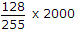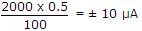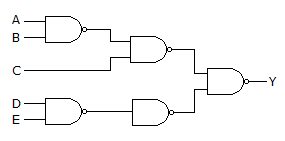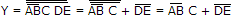# Electronics and Communication Engineering - Digital Electronics

16.

The access time of a word in 4 MB main memory is 100 ms. The access time of a word in a 32 kb data cache memory is 10 ns. The average data cache bit ratio is 0.95. The efficiency of memory access time is

 A. 9.5 ns B. 14.5 ns C. 20 ns D. 95 ns

Explanation:

Access time = 0.95 x 10 + 0.05 x 100.

17.

The expression Y = pM (0, 1, 3, 4) is

 A. POS B. SOP C. Hybrid D. none of the above

Explanation:

This is product of sums expression.

18.

An 8 bit DAC has a full scale output of 2 mA and full scale error of ± 0.5%. If input is 10000000 the range of outputs is

 A. 994 to 1014 μA B. 990 to 1020 μA C. 800 to 1200 μA D. none of the above

Explanation:

10000000 = 128, 11111111 = 255

If there is no error, output == 1004μA.

Maximum error =Hence range of output 994 to 1014 μA.

19.

Decimal 43 in hexadecimal and BCD number system is respectively.

 A. B2, 01000011 B. 2B, 01000011 C. 2B, 00110100 D. B2, 01000100

Explanation:

(43)10 = (2B)16

(43)10 = (01000011)2 .

20.

The circuit of the given figure realizes the functionA. Y = (A + B) C + DE B. Y = A + B + C + D + E C. AB + C +DE D. AB + C(D + E)

Explanation:or

Y = (A + B)C + DE.